Скачать презентацию Elasticity is a concept that relates

914752411e2194aa38f891606799cd81.ppt

• Количество слайдов: 35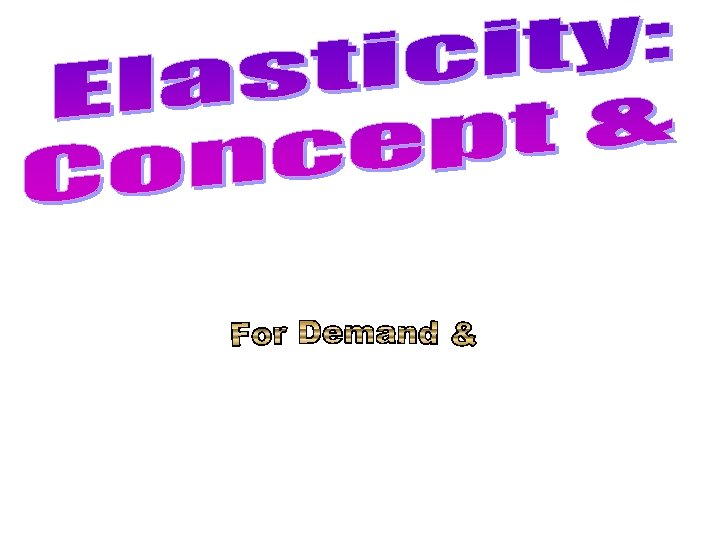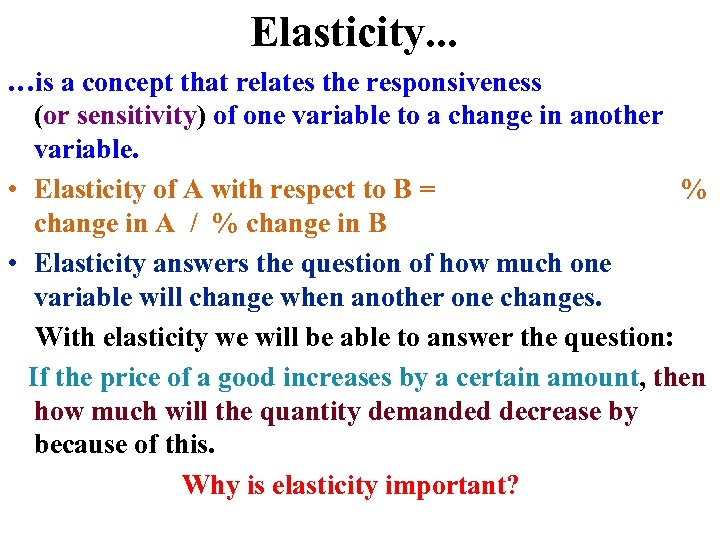Elasticity. . . …is a concept that relates the responsiveness (or sensitivity) of one variable to a change in another variable. • Elasticity of A with respect to B = % change in A / % change in B • Elasticity answers the question of how much one variable will change when another one changes. With elasticity we will be able to answer the question: If the price of a good increases by a certain amount, then how much will the quantity demanded decrease by because of this. Why is elasticity important?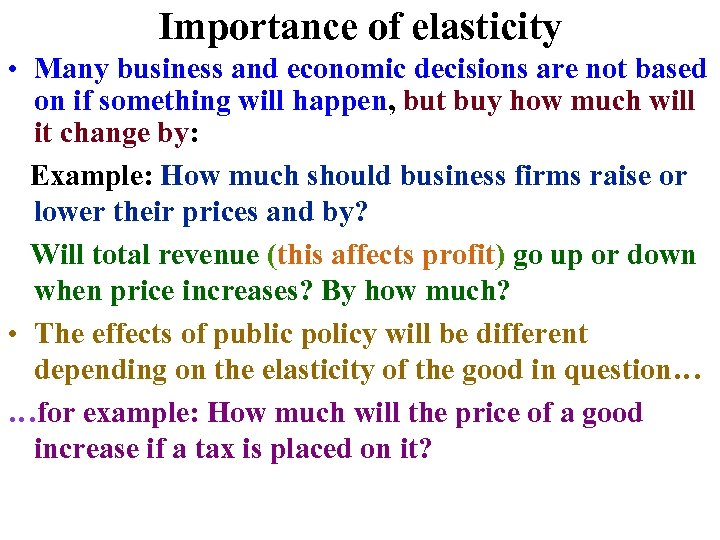Importance of elasticity • Many business and economic decisions are not based on if something will happen, but buy how much will it change by: Example: How much should business firms raise or lower their prices and by? Will total revenue (this affects profit) go up or down when price increases? By how much? • The effects of public policy will be different depending on the elasticity of the good in question… …for example: How much will the price of a good increase if a tax is placed on it?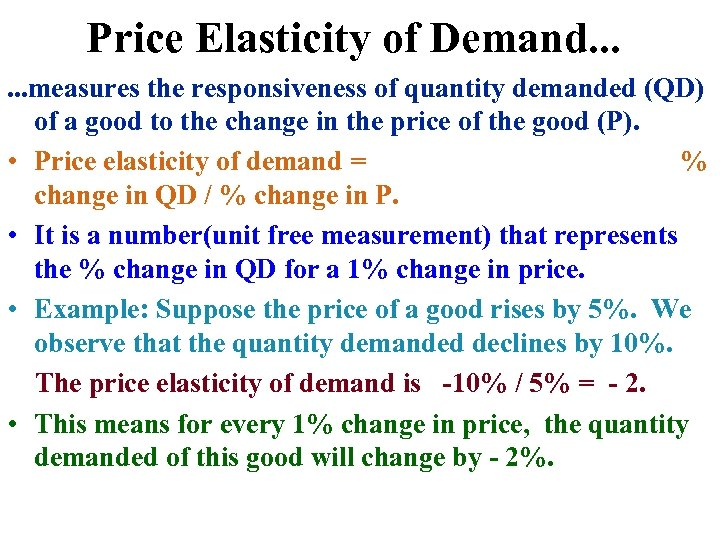Price Elasticity of Demand. . . measures the responsiveness of quantity demanded (QD) of a good to the change in the price of the good (P). • Price elasticity of demand = % change in QD / % change in P. • It is a number(unit free measurement) that represents the % change in QD for a 1% change in price. • Example: Suppose the price of a good rises by 5%. We observe that the quantity demanded declines by 10%. The price elasticity of demand is -10% / 5% = - 2. • This means for every 1% change in price, the quantity demanded of this good will change by - 2%.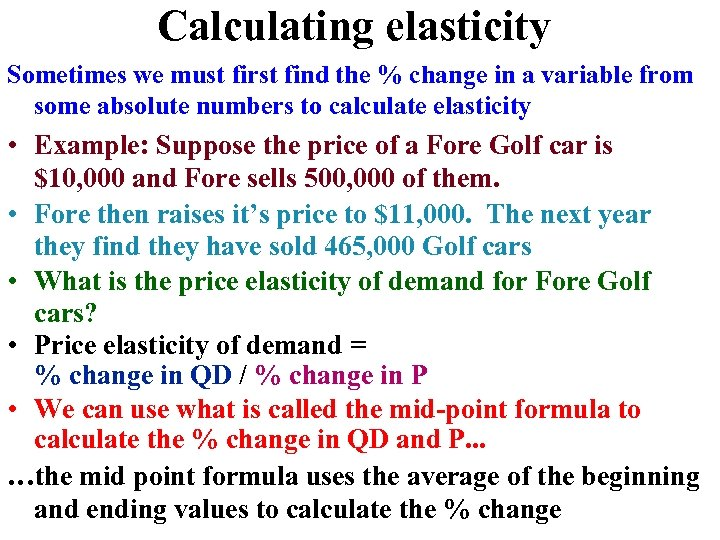Calculating elasticity Sometimes we must first find the % change in a variable from some absolute numbers to calculate elasticity • Example: Suppose the price of a Fore Golf car is \$10, 000 and Fore sells 500, 000 of them. • Fore then raises it’s price to \$11, 000. The next year they find they have sold 465, 000 Golf cars • What is the price elasticity of demand for Fore Golf cars? • Price elasticity of demand = % change in QD / % change in P • We can use what is called the mid-point formula to calculate the % change in QD and P. . . …the mid point formula uses the average of the beginning and ending values to calculate the % change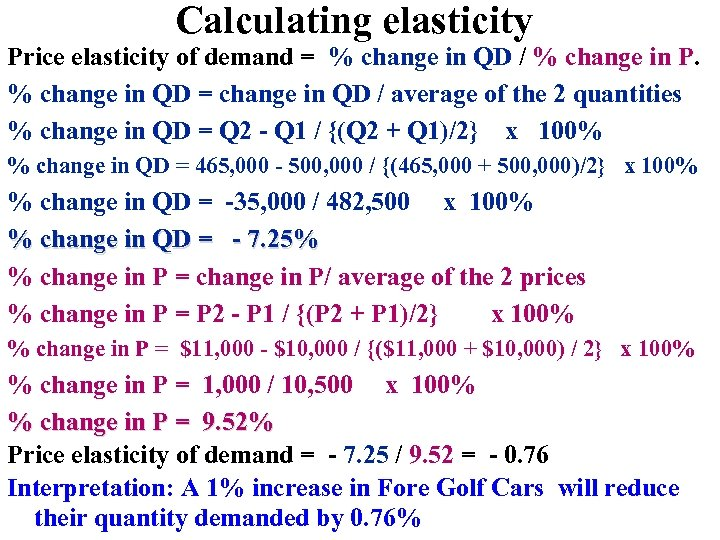Calculating elasticity Price elasticity of demand = % change in QD / % change in P. % change in QD = change in QD / average of the 2 quantities % change in QD = Q 2 - Q 1 / {(Q 2 + Q 1)/2} x 100% % change in QD = 465, 000 - 500, 000 / {(465, 000 + 500, 000)/2} x 100% % change in QD = -35, 000 / 482, 500 x 100% % change in QD = - 7. 25% % change in P = change in P/ average of the 2 prices % change in P = P 2 - P 1 / {(P 2 + P 1)/2} x 100% % change in P = \$11, 000 - \$10, 000 / {(\$11, 000 + \$10, 000) / 2} x 100% % change in P = 1, 000 / 10, 500 x 100% % change in P = 9. 52% Price elasticity of demand = - 7. 25 / 9. 52 = - 0. 76 Interpretation: A 1% increase in Fore Golf Cars will reduce their quantity demanded by 0. 76%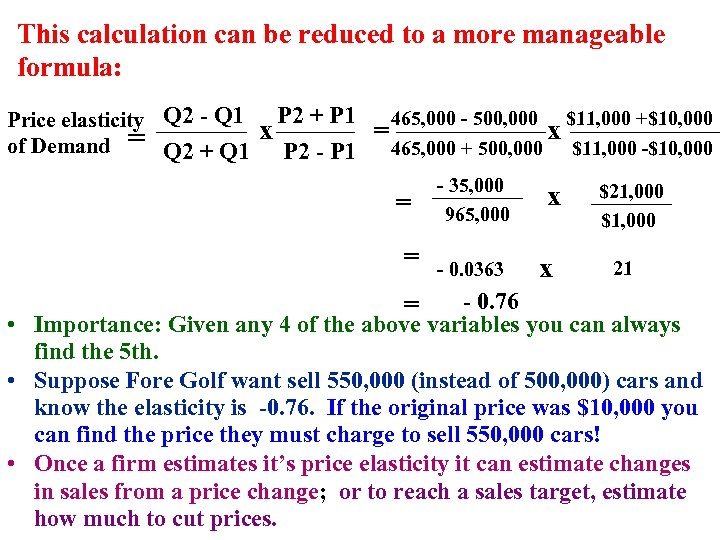This calculation can be reduced to a more manageable formula: Price elasticity Q 2 - Q 1 of Demand = Q 2 + Q 1 P 2 + P 1 465, 000 - 500, 000 \$11, 000 +\$10, 000 x = x P 2 - P 1 465, 000 + 500, 000 \$11, 000 -\$10, 000 = = - 35, 000 965, 000 - 0. 0363 x x \$21, 000 \$1, 000 21 - 0. 76 • Importance: Given any 4 of the above variables you can always find the 5 th. • Suppose Fore Golf want sell 550, 000 (instead of 500, 000) cars and know the elasticity is -0. 76. If the original price was \$10, 000 you can find the price they must charge to sell 550, 000 cars! • Once a firm estimates it’s price elasticity it can estimate changes in sales from a price change; or to reach a sales target, estimate how much to cut prices. =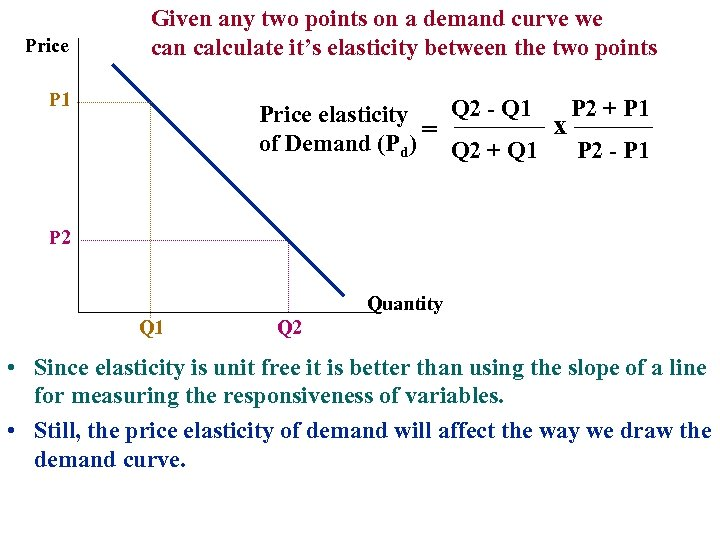Price Given any two points on a demand curve we can calculate it’s elasticity between the two points P 1 Q 2 - Q 1 P 2 + P 1 Price elasticity x = of Demand (Pd) Q 2 + Q 1 P 2 - P 1 P 2 Quantity Q 1 Q 2 • Since elasticity is unit free it is better than using the slope of a line for measuring the responsiveness of variables. • Still, the price elasticity of demand will affect the way we draw the demand curve.Types of Elasticity's • Economists group the numbers that are calculated into different types of elasticity depending on which percentage change is greater: price or quantity • Depending on the number that is calculated we put that number into 1 of 5 different types • We ignore the minus sign (use absolute value) when doing so.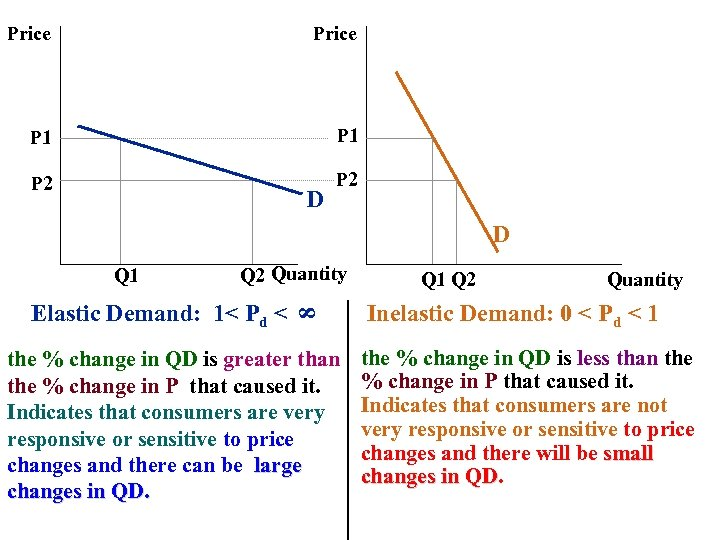Price P 1 P 2 D D Q 2 Quantity Elastic Demand: 1< Pd < 8 Q 1 the % change in QD is greater than the % change in P that caused it. Indicates that consumers are very responsive or sensitive to price changes and there can be large changes in QD. Q 1 Q 2 Quantity Inelastic Demand: 0 < Pd < 1 the % change in QD is less than the % change in P that caused it. Indicates that consumers are not very responsive or sensitive to price changes and there will be small changes in QD. QD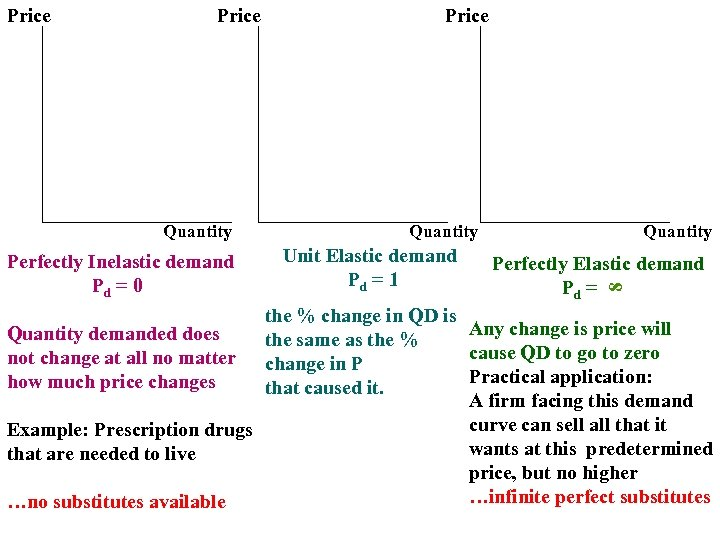Price Quantity Perfectly Inelastic demand Pd = 0 Price Quantity Unit Elastic demand Pd = 1 Quantity Perfectly Elastic demand Pd = 8 Price the % change in QD is Any change is price will Quantity demanded does the same as the % cause QD to go to zero not change at all no matter change in P Practical application: how much price changes that caused it. A firm facing this demand curve can sell all that it Example: Prescription drugs wants at this predetermined that are needed to live price, but no higher …infinite perfect substitutes …no substitutes available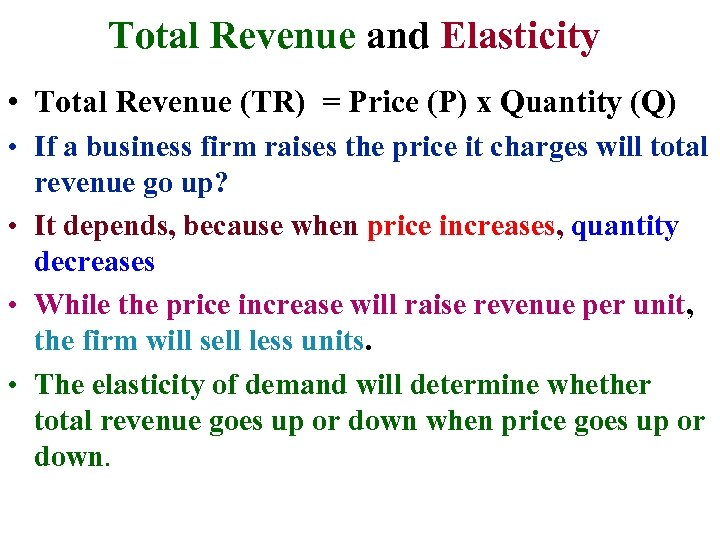Total Revenue and Elasticity • Total Revenue (TR) = Price (P) x Quantity (Q) • If a business firm raises the price it charges will total revenue go up? • It depends, because when price increases, quantity decreases • While the price increase will raise revenue per unit, the firm will sell less units. • The elasticity of demand will determine whether total revenue goes up or down when price goes up or down.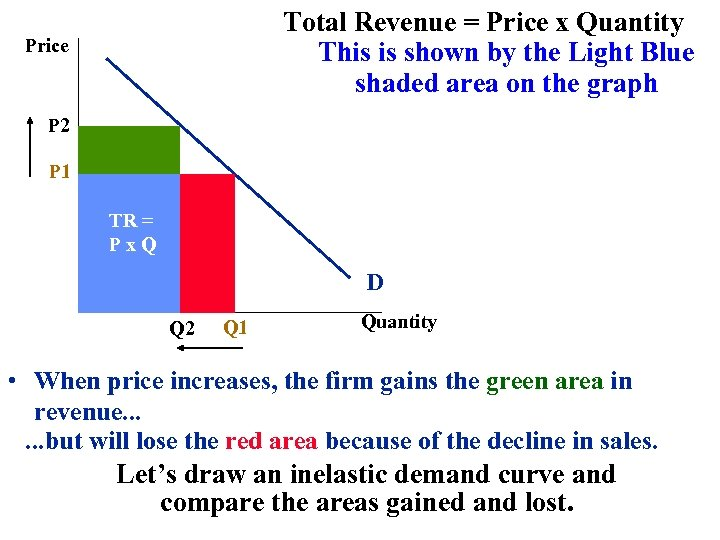Total Revenue = Price x Quantity This is shown by the Light Blue shaded area on the graph Price P 2 P 1 TR = Px. Q D Q 2 Q 1 Quantity • When price increases, the firm gains the green area in revenue. . . but will lose the red area because of the decline in sales. Let’s draw an inelastic demand curve and compare the areas gained and lost.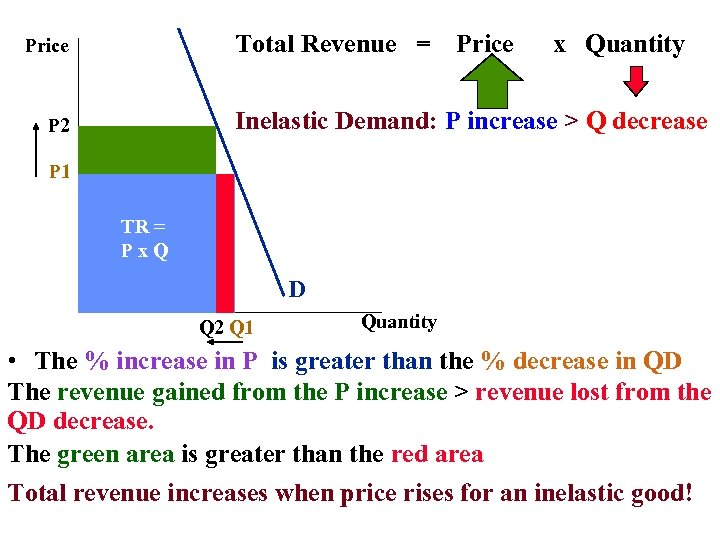Total Revenue = Price x Quantity Inelastic Demand: P increase > Q decrease P 2 P 1 TR = Px. Q D Q 2 Q 1 Quantity • The % increase in P is greater than the % decrease in QD The revenue gained from the P increase > revenue lost from the QD decrease. The green area is greater than the red area Total revenue increases when price rises for an inelastic good!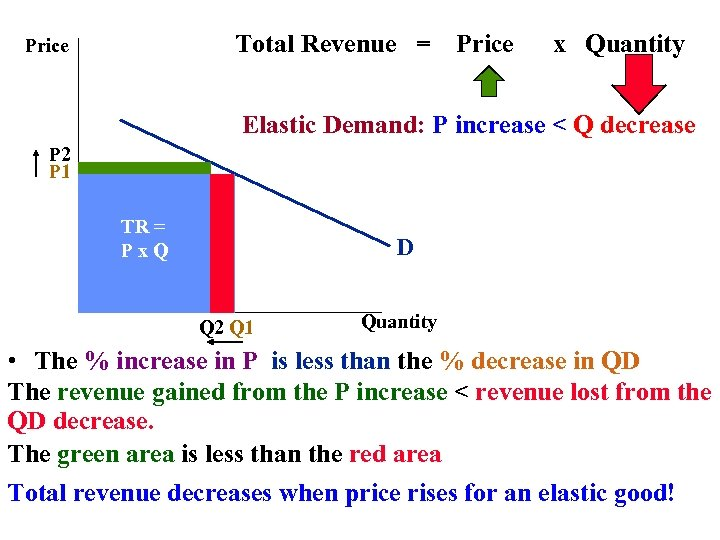Total Revenue = Price x Quantity Elastic Demand: P increase < Q decrease P 2 P 1 TR = Px. Q D Q 2 Q 1 Quantity • The % increase in P is less than the % decrease in QD The revenue gained from the P increase < revenue lost from the QD decrease. The green area is less than the red area Total revenue decreases when price rises for an elastic good!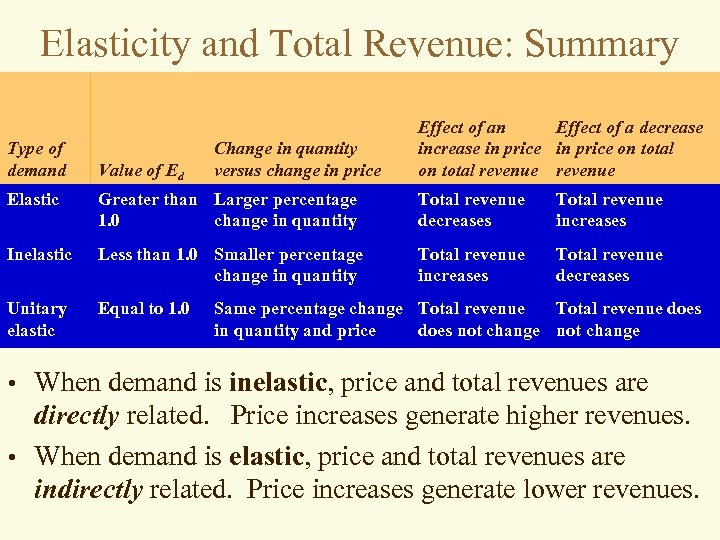Elasticity and Total Revenue: Summary Type of demand Value of Ed Change in quantity versus change in price Effect of an Effect of a decrease in price on total revenue Elastic Greater than Larger percentage 1. 0 change in quantity Total revenue decreases Total revenue increases Inelastic Less than 1. 0 Smaller percentage change in quantity Total revenue increases Total revenue decreases Unitary elastic Equal to 1. 0 Same percentage change Total revenue does in quantity and price does not change • When demand is inelastic, price and total revenues are directly related. Price increases generate higher revenues. • When demand is elastic, price and total revenues are indirectly related. Price increases generate lower revenues.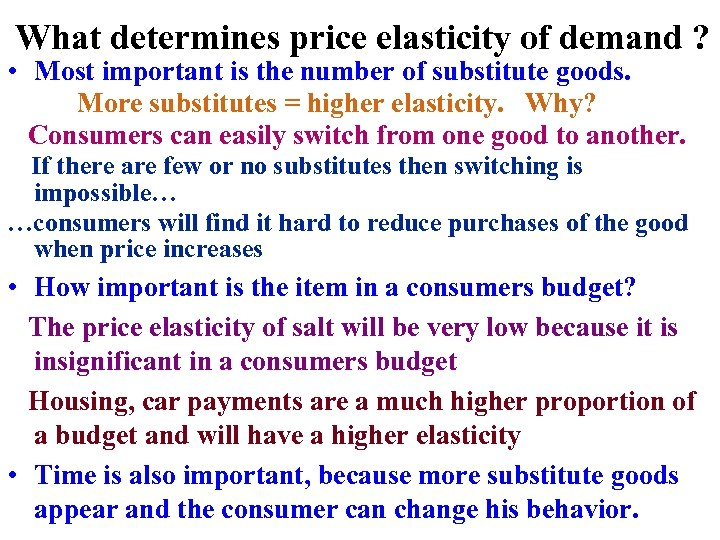What determines price elasticity of demand ? • Most important is the number of substitute goods. More substitutes = higher elasticity. Why? Consumers can easily switch from one good to another. If there are few or no substitutes then switching is impossible… …consumers will find it hard to reduce purchases of the good when price increases • How important is the item in a consumers budget? The price elasticity of salt will be very low because it is insignificant in a consumers budget Housing, car payments are a much higher proportion of a budget and will have a higher elasticity • Time is also important, because more substitute goods appear and the consumer can change his behavior.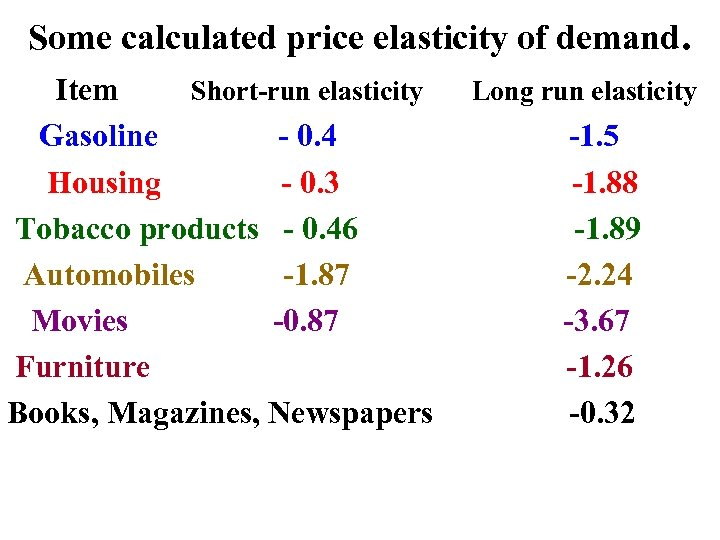Some calculated price elasticity of demand. Item Short-run elasticity Gasoline - 0. 4 Housing - 0. 3 Tobacco products - 0. 46 Automobiles -1. 87 Movies -0. 87 Furniture Books, Magazines, Newspapers Long run elasticity -1. 5 -1. 88 -1. 89 -2. 24 -3. 67 -1. 26 -0. 32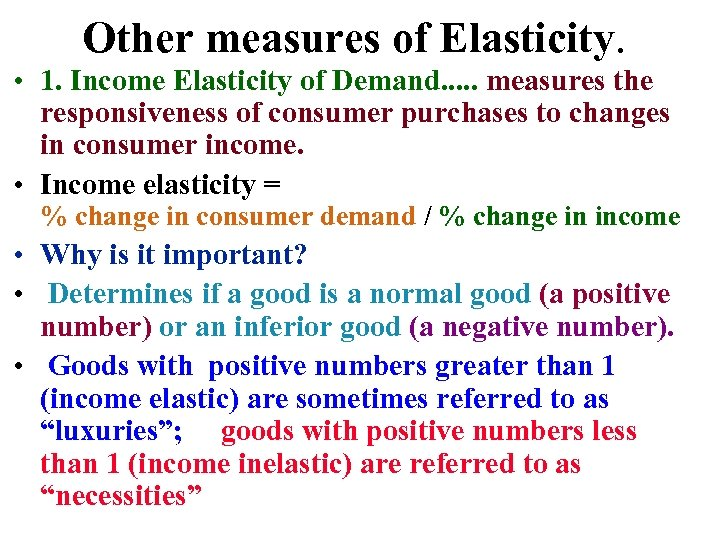Other measures of Elasticity. • 1. Income Elasticity of Demand. . . measures the responsiveness of consumer purchases to changes in consumer income. • Income elasticity = % change in consumer demand / % change in income • Why is it important? • Determines if a good is a normal good (a positive number) or an inferior good (a negative number). • Goods with positive numbers greater than 1 (income elastic) are sometimes referred to as “luxuries”; goods with positive numbers less than 1 (income inelastic) are referred to as “necessities”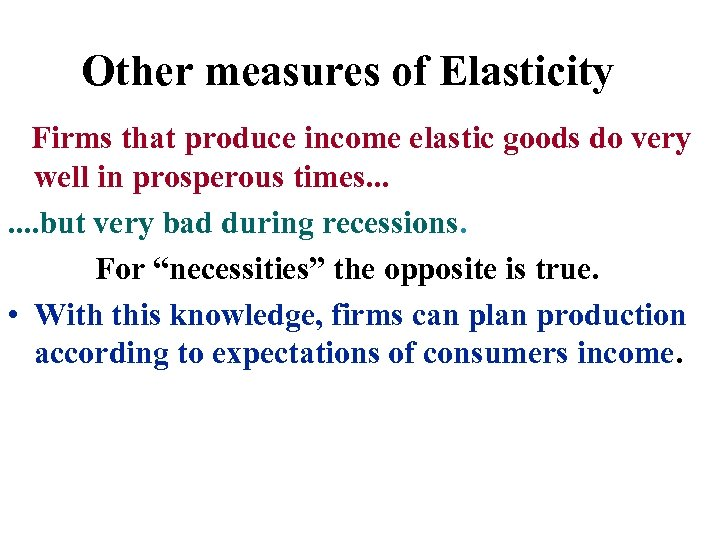Other measures of Elasticity Firms that produce income elastic goods do very well in prosperous times. . . . but very bad during recessions. For “necessities” the opposite is true. • With this knowledge, firms can plan production according to expectations of consumers income.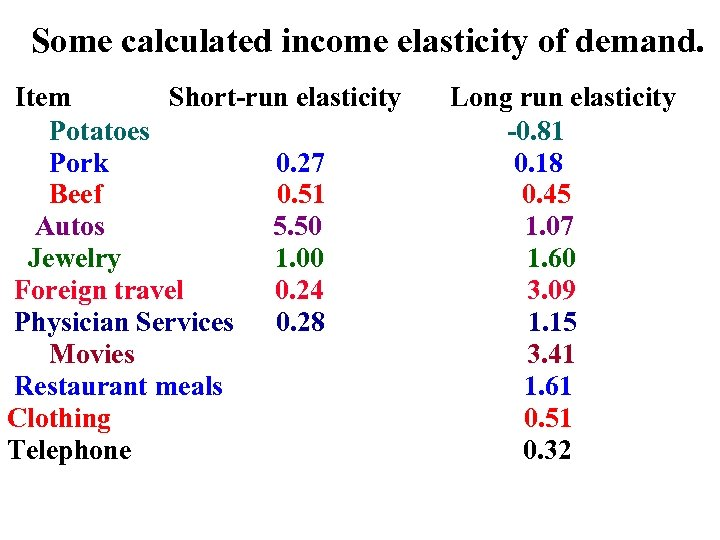Some calculated income elasticity of demand. Item Short-run elasticity Potatoes Pork 0. 27 Beef 0. 51 Autos 5. 50 Jewelry 1. 00 Foreign travel 0. 24 Physician Services 0. 28 Movies Restaurant meals Clothing Telephone Long run elasticity -0. 81 0. 18 0. 45 1. 07 1. 60 3. 09 1. 15 3. 41 1. 61 0. 51 0. 32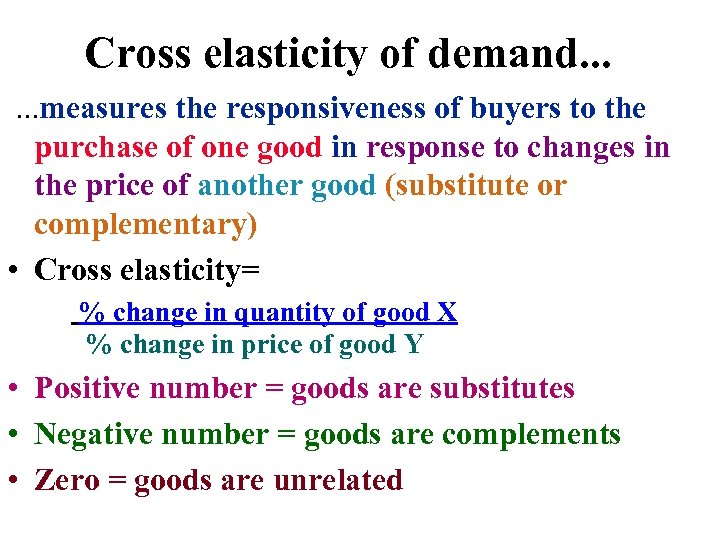Cross elasticity of demand. . . measures the responsiveness of buyers to the purchase of one good in response to changes in the price of another good (substitute or complementary) • Cross elasticity= % change in quantity of good X % change in price of good Y • Positive number = goods are substitutes • Negative number = goods are complements • Zero = goods are unrelated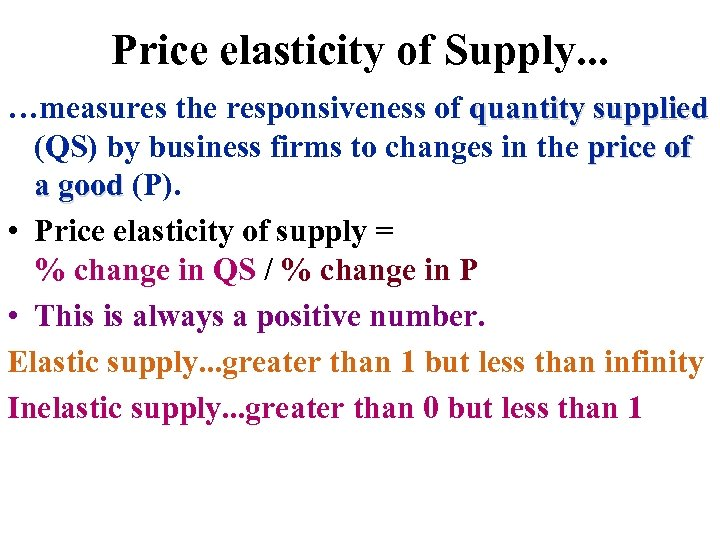Price elasticity of Supply. . . …measures the responsiveness of quantity supplied (QS) by business firms to changes in the price of a good (P). • Price elasticity of supply = % change in QS / % change in P • This is always a positive number. Elastic supply. . . greater than 1 but less than infinity Inelastic supply. . . greater than 0 but less than 1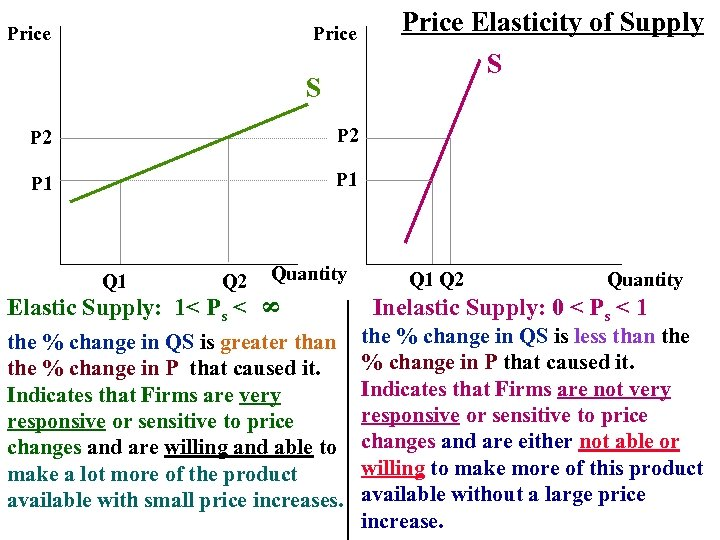Price S P 2 P 1 Price Elasticity of Supply S P 1 Q 2 Elastic Supply: 1< Ps < Quantity 8 Q 1 the % change in QS is greater than the % change in P that caused it. Indicates that Firms are very responsive or sensitive to price changes and are willing and able to make a lot more of the product available with small price increases. Q 1 Q 2 Quantity Inelastic Supply: 0 < Ps < 1 the % change in QS is less than the % change in P that caused it. Indicates that Firms are not very responsive or sensitive to price changes and are either not able or willing to make more of this product available without a large price increase.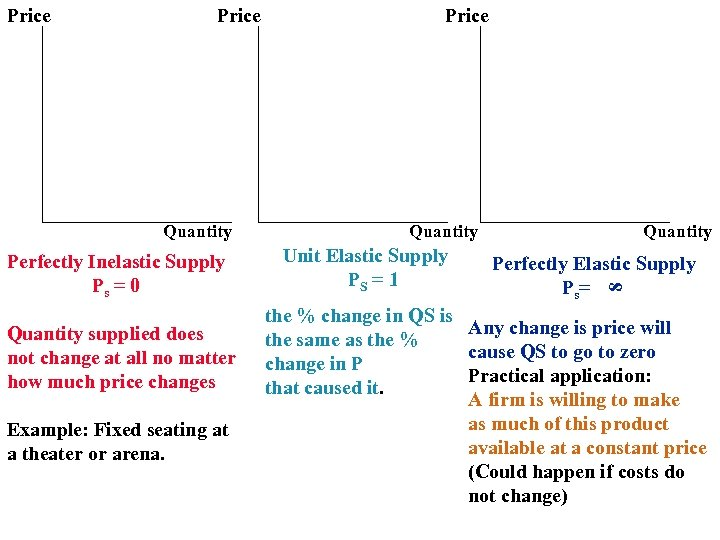Price Quantity Perfectly Inelastic Supply Ps = 0 Quantity supplied does not change at all no matter how much price changes Example: Fixed seating at a theater or arena. Price Quantity Unit Elastic Supply PS = 1 Quantity Perfectly Elastic Supply Ps= 8 Price the % change in QS is Any change is price will the same as the % cause QS to go to zero change in P Practical application: that caused it. A firm is willing to make as much of this product available at a constant price (Could happen if costs do not change)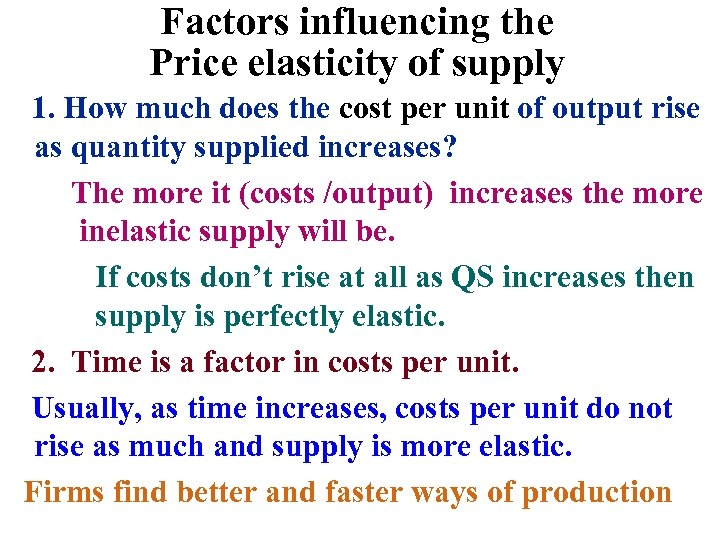Factors influencing the Price elasticity of supply 1. How much does the cost per unit of output rise as quantity supplied increases? The more it (costs /output) increases the more inelastic supply will be. If costs don’t rise at all as QS increases then supply is perfectly elastic. 2. Time is a factor in costs per unit. Usually, as time increases, costs per unit do not rise as much and supply is more elastic. Firms find better and faster ways of production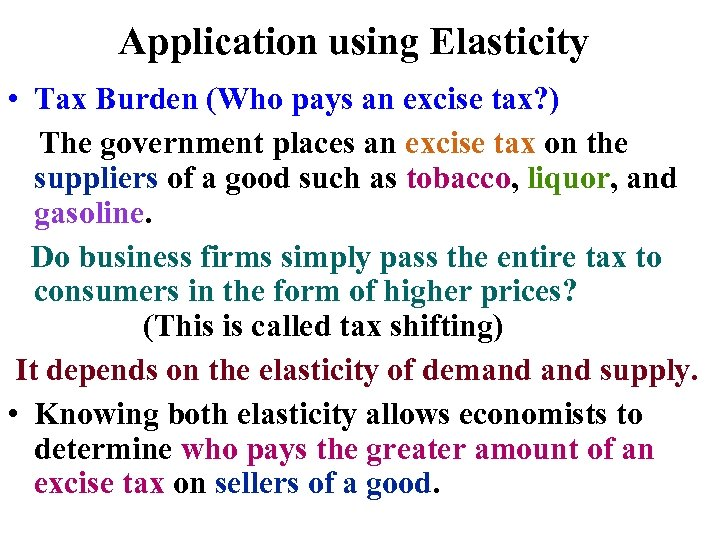Application using Elasticity • Tax Burden (Who pays an excise tax? ) The government places an excise tax on the suppliers of a good such as tobacco, liquor, and gasoline. Do business firms simply pass the entire tax to consumers in the form of higher prices? (This is called tax shifting) It depends on the elasticity of demand supply. • Knowing both elasticity allows economists to determine who pays the greater amount of an excise tax on sellers of a good.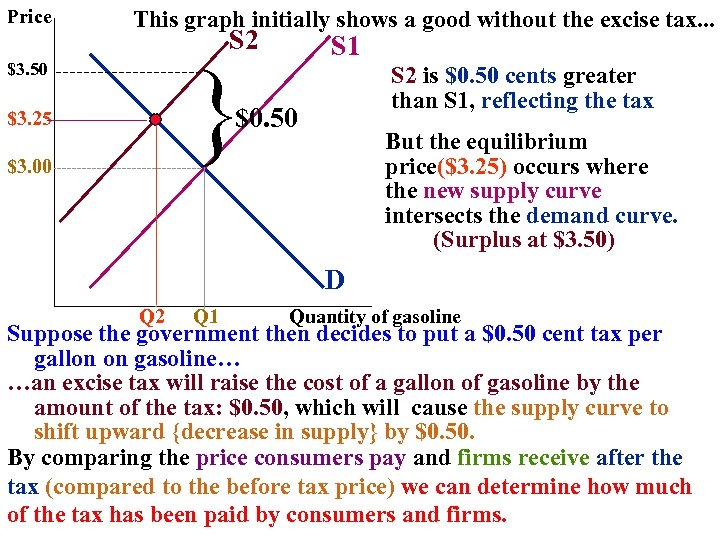Price This graph initially shows a good without the excise tax. . . S 2 } \$3. 50 S 1 S 2 is \$0. 50 cents greater than S 1, reflecting the tax \$0. 50 \$3. 25 \$3. 00 But the equilibrium price(\$3. 25) occurs where the new supply curve intersects the demand curve. (Surplus at \$3. 50) D Q 2 Q 1 Quantity of gasoline Suppose the government then decides to put a \$0. 50 cent tax per gallon on gasoline… …an excise tax will raise the cost of a gallon of gasoline by the amount of the tax: \$0. 50, which will cause the supply curve to shift upward {decrease in supply} by \$0. 50. By comparing the price consumers pay and firms receive after the tax (compared to the before tax price) we can determine how much of the tax has been paid by consumers and firms.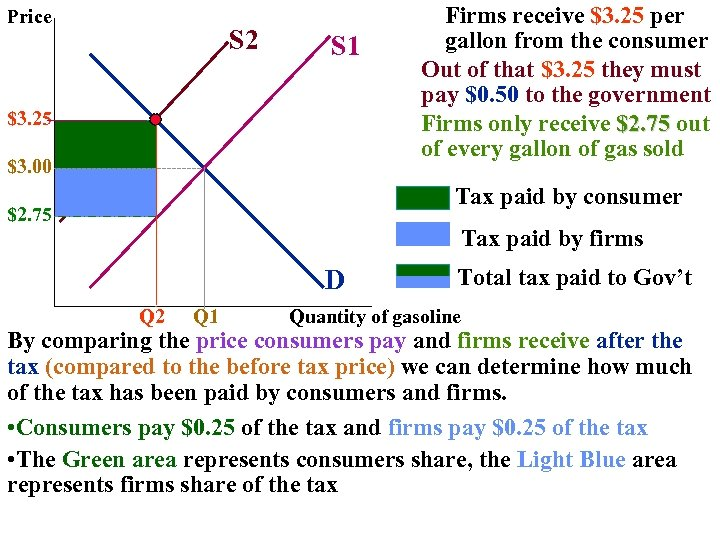Price S 2 S 1 \$3. 25 \$3. 00 Firms receive \$3. 25 per gallon from the consumer Out of that \$3. 25 they must pay \$0. 50 to the government Firms only receive \$2. 75 out of every gallon of gas sold Tax paid by consumer \$2. 75 Tax paid by firms D Q 2 Q 1 Total tax paid to Gov’t Quantity of gasoline By comparing the price consumers pay and firms receive after the tax (compared to the before tax price) we can determine how much of the tax has been paid by consumers and firms. • Consumers pay \$0. 25 of the tax and firms pay \$0. 25 of the tax • The Green area represents consumers share, the Light Blue area represents firms share of the tax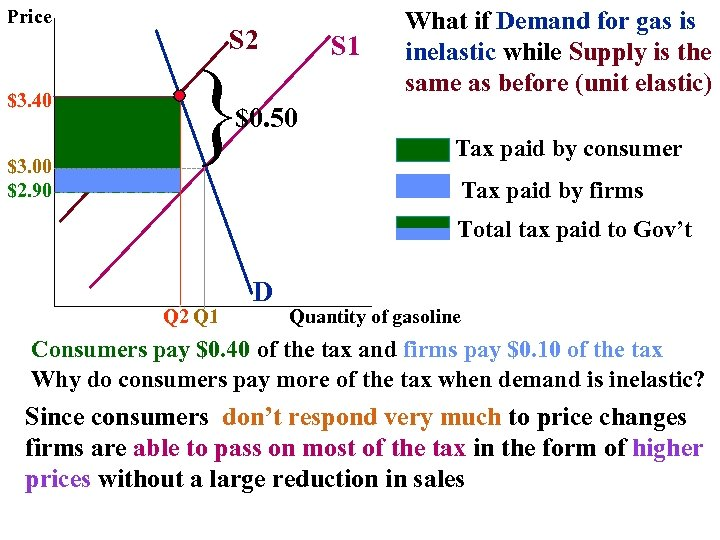Price \$3. 40 \$3. 00 \$2. 90 S 2 } S 1 What if Demand for gas is inelastic while Supply is the same as before (unit elastic) \$0. 50 Tax paid by consumer Tax paid by firms Total tax paid to Gov’t Q 2 Q 1 D Quantity of gasoline Consumers pay \$0. 40 of the tax and firms pay \$0. 10 of the tax Why do consumers pay more of the tax when demand is inelastic? Since consumers don’t respond very much to price changes firms are able to pass on most of the tax in the form of higher prices without a large reduction in sales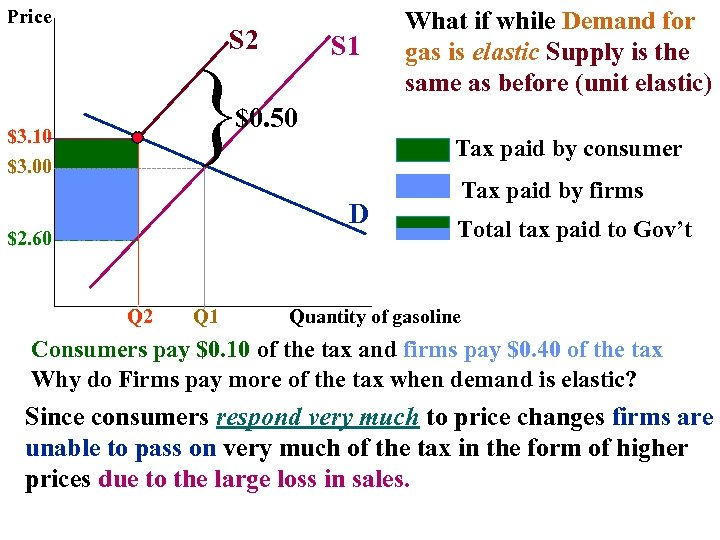Price S 2 } S 1 What if while Demand for gas is elastic Supply is the same as before (unit elastic) \$0. 50 \$3. 10 \$3. 00 Tax paid by consumer D \$2. 60 Q 2 Q 1 Tax paid by firms Total tax paid to Gov’t Quantity of gasoline Consumers pay \$0. 10 of the tax and firms pay \$0. 40 of the tax Why do Firms pay more of the tax when demand is elastic? Since consumers respond very much to price changes firms are unable to pass on very much of the tax in the form of higher prices due to the large loss in sales.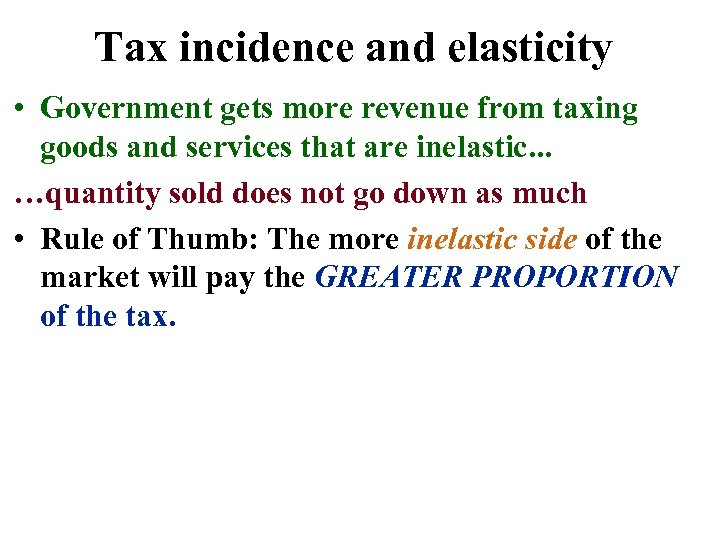Tax incidence and elasticity • Government gets more revenue from taxing goods and services that are inelastic. . . …quantity sold does not go down as much • Rule of Thumb: The more inelastic side of the market will pay the GREATER PROPORTION of the tax.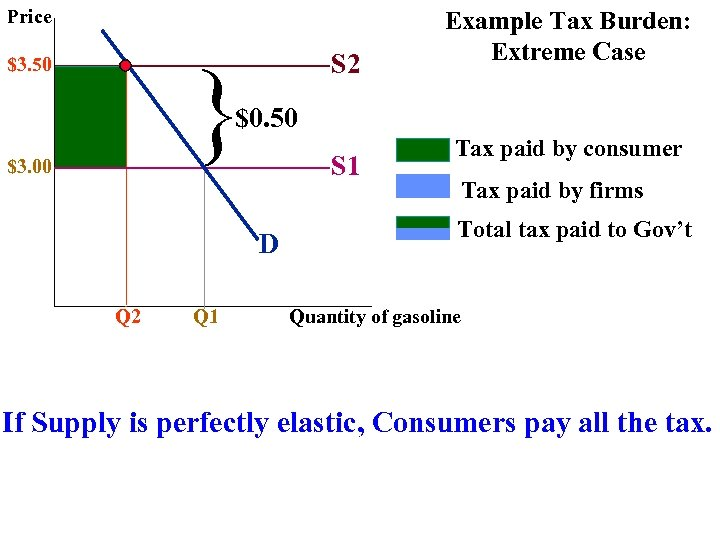Price } \$3. 50 S 2 Example Tax Burden: Extreme Case \$0. 50 \$3. 00 S 1 D Q 2 Q 1 Tax paid by consumer Tax paid by firms Total tax paid to Gov’t Quantity of gasoline If Supply is perfectly elastic, Consumers pay all the tax.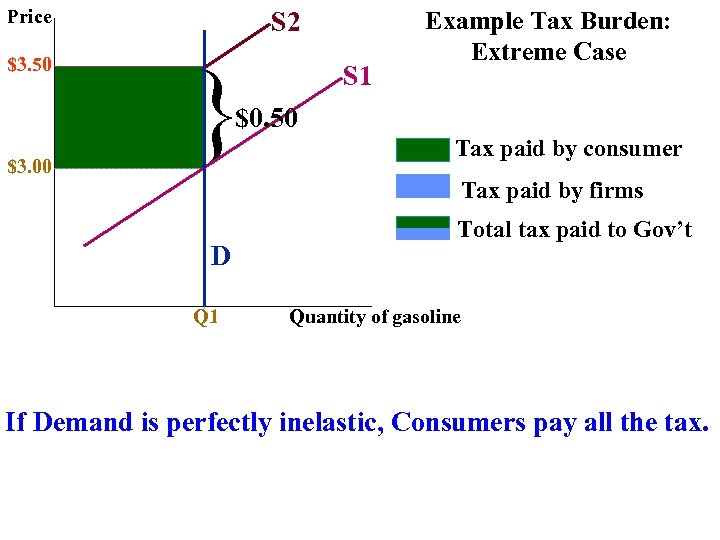Price \$3. 50 S 2 } S 1 Example Tax Burden: Extreme Case \$0. 50 \$3. 00 Tax paid by consumer Tax paid by firms D Q 1 Total tax paid to Gov’t Quantity of gasoline If Demand is perfectly inelastic, Consumers pay all the tax.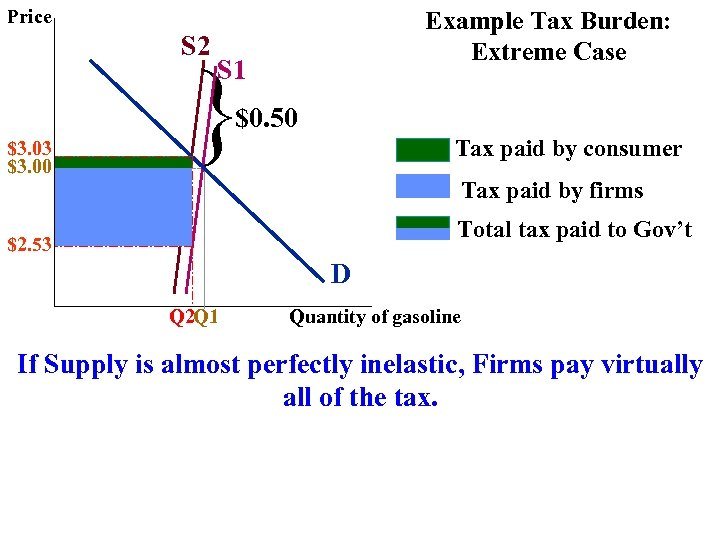Price S 2 Example Tax Burden: Extreme Case } S 1 \$0. 50 \$3. 03 \$3. 00 Tax paid by consumer Tax paid by firms Total tax paid to Gov’t \$2. 53 D Q 2 Q 1 Quantity of gasoline If Supply is almost perfectly inelastic, Firms pay virtually all of the tax.